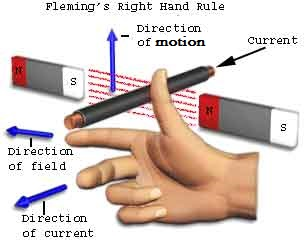# Motional EMF from Faraday's Laws and Fleming's Right Hand Rule | cbse24.com

### Motional emf from Faraday's law:

Induced emf by the change of the area of the coil linked with the magnetic field. The emf induced across the ends of a conductor due to its motion in a magnetic field is called motional emf. As shown in fig consider a conductor PQ of length l free to move on U-shaped conducting rails situated in a uniform and time-independent magnetic field B, directed normally into the plane of the paper. The conductor PQ is moved inwards with a speed v.As the conductor slide towards left, the area of the regular loop PQRS decrease. This decreases the magnetic flux linked with the closed-loop. Hence an emf is set up across the ends of conductor PQ because of which an induced current can be determined by using Fleming's right-hand rule.Induced current by changing area of the rectangular loop

Suppose a length x of the loop lies inside the magnetic field at any instant of time t. Then the magnetic flux linked with the rectangular loop PQRS is

ϕ=B.A=Blx

According to Faraday's law of electromagnetic induction , the induced emf is

e=dϕdt=d(Blx)dy=Bldxdt

e=Blv

Where dxdt=v

because the velocity v is in the decreasing direction of x.The induced emf Blv is called motional emf because this emf is induced due to the motion of a conductor in a magnetic field.

### Fleming's Right-Hand Rule:

This rule gives the direction of the induced current set up in a conductor moving perpendicular magnetic field.

If we stretch the thumb and the first two figures of our right hand in mutually perpendicular and if the forefinger points in the direction of the magnetic field , thumb in the direction of motion of the conductor, then the central figure points in the direction of current induced in the conductor.Fleming's right-hand rule

*